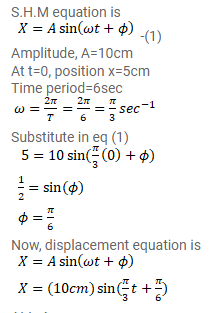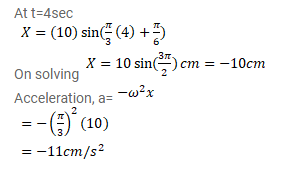# A particle executes simple harmonic motion with an amplitude of

Question:

A particle executes simple harmonic motion with an amplitude of $10 \mathrm{~cm}$ and time period $6 \mathrm{~s}$. At $t=0$ it is at position $x=5 \mathrm{~m}$ going towards positive $x$-direction. Write the equation for the displacement $x$ at time $t$. Find the magnitude of the acceleration of the particle at $t=4 \mathrm{~s}$.

Solution: